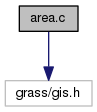GRASS Programmer's Manual  6.5.svn(2014)-r66266
gis/area.c File Reference
`#include <grass/gis.h>`
Include dependency graph for gis/area.c:Go to the source code of this file.

## Functions

int G_begin_cell_area_calculations (void)
Begin cell area calculations. More...

double G_area_of_cell_at_row (int row)
Cell area in specified row. More...

int G_begin_polygon_area_calculations (void)
Begin polygon area calculations. More...

double G_area_of_polygon (const double *x, const double *y, int n)
Area in square meters of polygon. More...

## Function Documentation

 double G_area_of_cell_at_row ( int row )

Cell area in specified row.

This routine returns the area in square meters of a cell in the specified row. This value is constant for planimetric grids and varies with the row if the projection is latitude-longitude.

Parameters
 [in] row
Returns
double

Definition at line 91 of file gis/area.c.

References tools::window.

Referenced by N_init_geom_data_2d().

 double G_area_of_polygon ( const double * x, const double * y, int n )

Area in square meters of polygon.

Returns the area in square meters of the polygon described by the n pairs of x,y coordinate vertices. It is used both for planimetric and latitude-longitude projections.
Returns the area in coordinate units of the polygon described by the n pairs of x,y coordinate vertices for planimetric grids. If the units for x,y are meters, then the area is in square meters. If the units are feet, then the area is in square feet, and so on.
Note: If the database is planimetric with the non-meter grid, this routine performs the required unit conversion to produce square meters.

Parameters
 [in] x array of x coordinates [in] y array of y coordinates [in] n number of x,y coordinate pairs
Returns
area in coordinate units of the polygon

Definition at line 165 of file gis/area.c.

References G_ellipsoid_polygon_area(), and G_planimetric_polygon_area().

Referenced by Vect_find_island(), Vect_get_area_area(), and Vect_isle_find_area().

 int G_begin_cell_area_calculations ( void )

Begin cell area calculations.

This routine must be called once before any call to G_area_of_cell_at_row(). It perform all inititalizations needed to do area calculations for grid cells, based on the current window "projection" field. It can be used in either planimetric projections or the latitude-longitude projection.
If the return value is 1 or 0, all the grid cells in the map have the same area. Otherwise, the area of a grid cell varies with the row.

Returns
0 if the projection is not measurable (ie. imagery or xy)
1 if the projection is planimetric (ie. UTM or SP)
2 if the projection is non-planimetric (ie. latitude-longitude)

Definition at line 50 of file gis/area.c.

Referenced by N_init_geom_data_2d().

 int G_begin_polygon_area_calculations ( void )

Begin polygon area calculations.

This initializes the polygon area calculation routines. It is used both for planimetric and latitude-longitude projections.

Returns
0 if the projection is not measurable (ie. imagery or xy)
1 if the projection is planimetric (ie. UTM or SP)
2 if the projection is non-planimetric (ie. latitude-longitude)

Definition at line 123 of file gis/area.c.

Referenced by Vect_find_island(), Vect_get_area_area(), and Vect_isle_find_area().